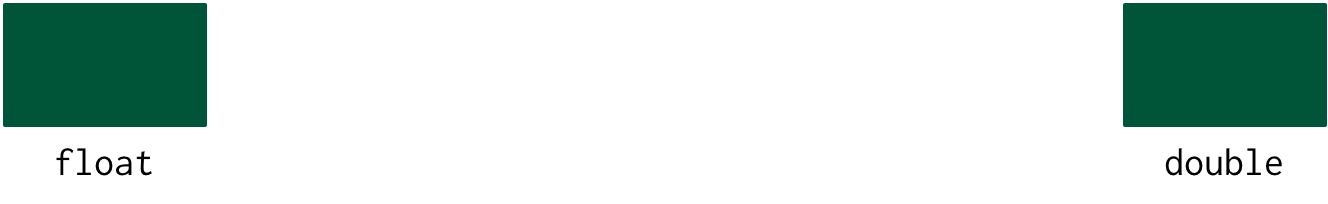# Primitive Types

TODO – NEED TO TALK ABOUT bind-val TODO – Equality and Types

In this chapter we will introduce xtlang’s type system. Unlike Scheme and other dynamically typed languages (e.g. Ruby and Python), XTLang requires you to define the types of all the variables that you are using. If you do not define the variables properly then the compiler will reject it and give you a (hopefully) helpful message about what needs to be fixed.

``````(bind-func will-not-compile
(lambda (x y)
(+ x y)))
``````

If you try to compile the source code above you will get an error as the compiler has no way of knowing whether x and y should be integers, floats, or doubles. Instead we need to define their types using type annotations:

``````(bind-func will-compile
(lambda (x:i32 y:i32)
(+ x y)))
``````

It would be tedious if you had to provide type annotations for every variable. Fortunately Extempore will always infer the correct type for a type if it has enough information:

``````(bind-func will-compile
(lambda (x:i32 y)
(+ x y)))
``````

So what about those situations where you have two variables of different types that need to be combined? XTLang provides the `convert` function for these situations:

``````(bind-func will-compile
(lambda (x:i32 y:float)
(+ x (convert y))))
``````

We will discuss in a later chapter why this works, but for the moment it is enough to know that `convert` will automagically convert types for you.

Extempore has four categories of types:

• Primitive Value Types (e.g. variables that contain integers, or floating point numbers)
• Memory Pointers (i.e. a variable that contains a memory location)
• User Defined Types
• Closure Types (i.e. the type signature of a function)

## Primitive Value Types

FOOTNOTE: If you’re a C programmer these are essentially the same as the C types. Each type is low level and represent a particular bit pattern in memory —there’s no boxing or unboxing going on. All types in Extempore are signed.

### Booleans

XTLang doesn’t have an explicit boolean type. Instead it stores booleans in an integer type with a a size of 1 bit: - `i1` (boolean)

‘0’ represents ‘False’ and ‘1’ represents ‘True’.

``````(bind-val true i1 1)
(bind-val false i1 0)
``````

### Characters

XTLang doesn’t have an explicit character type. Instead characters are stored using an integer type that has the size of 1 byte, or 8 bits: - `i8` (char)

``````(bind-val ch i8 'c')
``````

Note: The primitive character type only supports ASCII characters. TODO UTF support?

### Integers

XTLang only supports signed integers. XTLang supports the following sizes of integers:

• `i1` (boolean)
• `i8` (char)
• `i32`
• `i64` (default)

By default all integers in XTLang are 64 bit. Which means you can write the following code without the compiler complaining:

``````(bind-func very-simple-func
(lambda ()
34))
``````

as the compiler knows that 34 should be treated as a type of `i64`.

Note that the compiler will only treat 34 as a type of i64 if there is no conflicting type information. In this code 34 is treated as an i32:

``````(bind-func very-simple-func
(lambda (x:i32)
(+ x 34)))
``````

### Floating Point NumbersThere are two sizes of floating point number in XTLang:

• `float` (32 bit)
• `double` (64 bit, default)

Float literals in xtlang code (e.g. `4.2`) are interpreted as `double`:

``````(bind-func very-simple-func2
(lambda ()
34.3))
``````

unless the type signatures suggest otherwise:

``````(bind-func very-simple-func
(lambda (x:float)
(+ x 34.3)))
``````

### Strings

[fn:: Extempore also supports C style strings. These are discussed in the chapter on C interop]. Need something on Strings to go here.

## Memory Pointer Types

A memory pointer is a type whose value is the memory address of a value stored somewhere in the memory of your computer. For example:

• `double*` - the memory address of a double value.
• `i64*` - the memory address of an i64 value.

A pointer type are indicated by adding a `*` to the end of the base type. For example:

• `float` - A float value.
• `float*` - a pointer to a float value.
• `float**` - a pointer to a pointer to a float object.

[fn:: Unlike in C, `*` is not a dereference operator, it’s just the syntax for the specifying pointer types.]

You can get the memory location for a value using pref-ptr:

If you have a memory pointer you can access the value contained within it by using `pref`. `pref` takes two values: a memory pointer and an index.

``````(bind-func get-val
(lambda (a:i64*)
(printf "%p" (pref a 0))))
``````

In this example we are accessing the `i64` value stored at t he memory location contained in `a`. We could also access the value in the memory locations that come after `a`. For example:

``````(bind-func get-val-2-after
(lambda (a:i64*)
(printf "%p" (pref a 2))))
``````

This function is accessing the value in the location two elements after `a`. Don’t worry if this isn’t clear, we will discuss this in greater detail in future chapters. For the moment it is enough that you understand what a pointer is, and that you can recognize the type.

[fn:: If you’re a C programmer you’re probably wondering about pointer arithmetic. Extempore always increments by the number of bytes used to store your type. E.g. for an i32 it will always increment by four bytes at a time, while for an i64 it will increment by 8 bytes.]